# Mechanical Engineering - Hydraulics and Fluid Mechanics

### Exercise :: Hydraulics and Fluid Mechanics - Section 1

6.

The diameter of the nozzle (d) for maximum transmission of power is given by (where D = Diameter of pipe, f = Darcy 's coefficient of friction for pipe, and l = Length of pipe )

 A.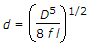B.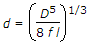C.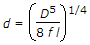D.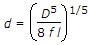Explanation:

No answer description available for this question. Let us discuss.

7.

The discharge over a right angled notch is (where H = Height of liquid above the apex of notch)

 A.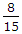Cd 2g x H B.Cd 2g x H3/2 C.Cd 2g x H2 D.Cd 2g x H5/2

Explanation:

No answer description available for this question. Let us discuss.

8.

A weir is said to be broad crested weir, if the width of the crest of the weir is __________ half the height of water above the weir crest.

 A. equal to. B. less than C. more than

Explanation:

No answer description available for this question. Let us discuss.

9.

A vertical wall is subjected to a pressure due to one kind of liquid, on one of its sides. The total pressure on the wall per unit length is (where w = Specific weight of liquid, and H = Height of liquid)

 A. wH B. wH/2 C. wH2/2 D. wH2/3

Explanation:

No answer description available for this question. Let us discuss.

10.

An error of 1% in measuring head over the apex of the notch (H) will produce an error of __________ in discharge over a triangular notch.

 A. 1% B. 1.5% C. 2% D. 2.5%